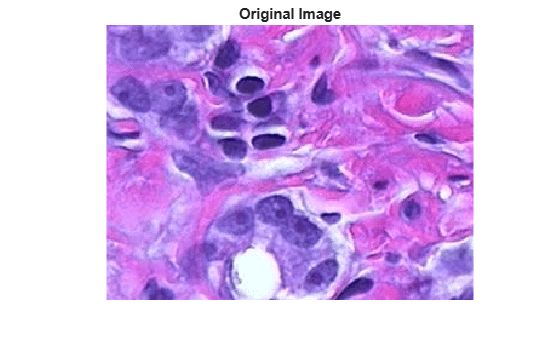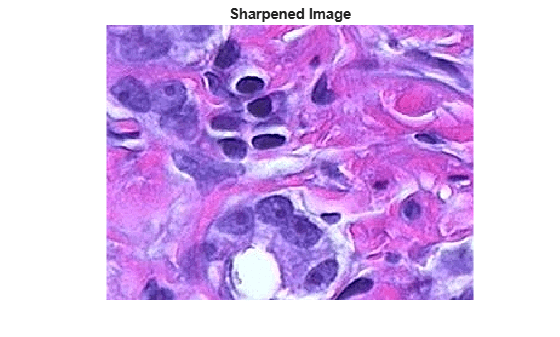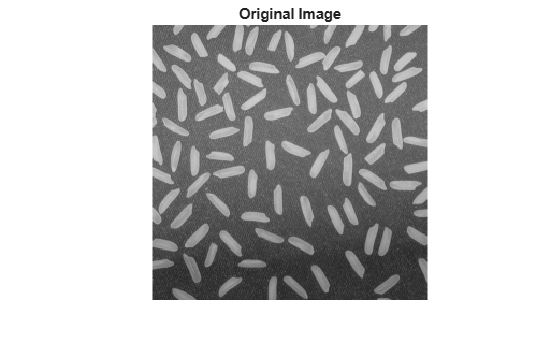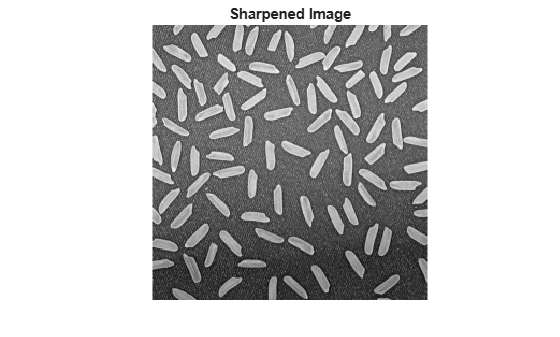# imsharpen

## Syntax

``B = imsharpen(A)``
``B = imsharpen(A,Name,Value)``

## Description

example

````B = imsharpen(A)` sharpens the grayscale or truecolor (RGB) image `A` by using the unsharp masking method.```

example

````B = imsharpen(A,Name,Value)` uses name-value arguments to control aspects of the unsharp masking.```

## Examples

collapse all

Read an image into the workspace and display it.

```a = imread('hestain.png'); imshow(a) title('Original Image');```Sharpen the image using the `imsharpen` function and display it.

```b = imsharpen(a); figure, imshow(b) title('Sharpened Image');```Read an image into the workspace and display it.

```a = imread('rice.png'); imshow(a), title('Original Image');```Sharpen image, specifying the `radius` and `amount` parameters.

```b = imsharpen(a,'Radius',2,'Amount',1); figure, imshow(b) title('Sharpened Image');```## Input Arguments

collapse all

Image to be sharpened, specified as a grayscale or RGB image.

Data Types: `single` | `double` | `int8` | `int16` | `int32` | `uint8` | `uint16` | `uint32`

### Name-Value Arguments

Specify optional pairs of arguments as `Name1=Value1,...,NameN=ValueN`, where `Name` is the argument name and `Value` is the corresponding value. Name-value arguments must appear after other arguments, but the order of the pairs does not matter.

Example: `B = imsharpen(A,Radius=1.5);` performs sharpening using a Gaussian lowpass filter with standard deviation `1.5`.

Before R2021a, use commas to separate each name and value, and enclose `Name` in quotes.

Example: ```B = imsharpen(A,"Radius",1.5);```

Standard deviation of the Gaussian lowpass filter, specified as a positive number. This argument controls the size of the region around the edge pixels that is affected by sharpening. A large value sharpens wider regions around the edges, whereas a small value sharpens narrower regions around edges.

Example: `Radius=1.5`

Data Types: `single` | `double` | `int8` | `int16` | `int32` | `int64` | `uint8` | `uint16` | `uint32` | `uint64`

Strength of the sharpening effect, specified as a number. A larger value leads to larger increase in the contrast of the sharpened pixels. Typical values for this parameter are within the range [0, 2], although values greater than `2` are allowed. Very large values for this argument may create undesirable effects in the output image.

Example: `Amount=1.2`

Data Types: `single` | `double` | `int8` | `int16` | `int32` | `int64` | `uint8` | `uint16` | `uint32` | `uint64`

Minimum contrast required for a pixel to be considered an edge pixel, specified as a number in the range [0, 1]. Larger values (closer to 1) allow sharpening only in high-contrast regions, such as strong edges, while leaving low-contrast regions unaffected. Smaller values (closer to 0) additionally allow sharpening in relatively smoother regions of the image. This argument is useful in avoiding sharpening noise in the output image.

Example: `Threshold=0.7`

Data Types: `single` | `double` | `int8` | `int16` | `int32` | `int64` | `uint8` | `uint16` | `uint32` | `uint64`

## Output Arguments

collapse all

Sharpened image, returned as a numeric array of the same size and data type as the input image `A`.

collapse all

### Sharpening

Sharpness is the contrast between different colors. A quick transition from black to white looks sharp. A gradual transition from black to gray to white looks blurry. Sharpening images increases the contrast along the edges where different colors meet.

The unsharp masking technique comes from a publishing industry process in which an image is sharpened by subtracting a blurred (unsharp) version of the image from itself. Do not be confused by the name of this filter: an unsharp filter is an operator used to sharpen an image.

## Tips

• If `A` is a truecolor (RGB) image, then `imsharpen` converts the image to the L*a*b* color space, applies sharpening to the L* channel only, and then converts the image back to the RGB color space before returning it as the output image `B`.

## Version History

Introduced in R2013a

expand all